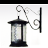# PSoC™ Creator & Designer Forum DiscussionsLevel 2
Level 2# Float is changing the actual value

Hi,

I'm trying to get first decimal from a float, however when I'm debigging the aplication it changes the actual value for exmaple:

float test = 5.1;

The debugger says that the value stored is 5.0999999, the same issue is happenning with

float test = 5.100001;

debugger says that test = 5.100004.

With this issue I can't get the fist decimal.

Any idea??

1 SolutionLevel 10
Level 10# Re: Float is changing the actual value

The difference you see has to do with the representation of floats as digital numbers, you will always get divergences between decimal and float representations.

What exactly do you need the first decimal for? When you want to display that number use sprintf()

You even may use

HelpInt = (int)(fabs(MyFloat) * 10.0);

Help2Int = HelpInt / 10;

Help2Int *= 10;

FirstDec = HelpInt - Help2Int;

Another way could be to add 0.05 to force rounding

See a discussion about round() here.

Happy coding

BobLevel 10
Level 10# Re: Float is changing the actual value

The difference you see has to do with the representation of floats as digital numbers, you will always get divergences between decimal and float representations.

What exactly do you need the first decimal for? When you want to display that number use sprintf()

You even may use

HelpInt = (int)(fabs(MyFloat) * 10.0);

Help2Int = HelpInt / 10;

Help2Int *= 10;

FirstDec = HelpInt - Help2Int;

Another way could be to add 0.05 to force rounding

See a discussion about round() here.

Happy coding

Bob